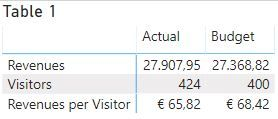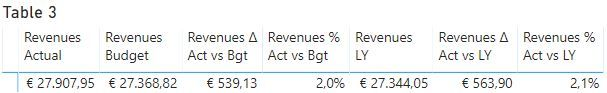cancel
Showing results for
Did you mean:

Fabric is Generally Available. Browse Fabric Presentations. Work towards your Fabric certification with the Cloud Skills Challenge.Regular Visitor

## Combine measures in Matrix visual and calculate variance by date and period

Dear datanauts,

I am trying to combine measures in a matrix split by date and period (Actual and Budget).

You can access my files here (please take a copy locally):

https://www.dropbox.com/sh/x2rzap374vven36/AACA94W9ZdKosUEbleaoZ-4ja?dl=0

Excel Report.jpg

is what I am trying to reproduce from Excel to Power BIexample.xlsx

is my data source excel file

example.pbix

is my power bi model

example.xlsx contents

Sheet "Data"

One table named Financials which contains Revenues and Expenses split by month and period.

Another table named Operationals which contains number of visitors split by month and period.

Sheet "Calendar"

A table with reported Dates

Sheet "Period"

A table with a split of periods I need to report (Actual, Budget and Last Year)

Sheet "Report"

The report I am trying to reproduce from Excel to Power BI

I have created the following model in Power BIMeasures Approach 1

I have created the following measures

Revenues = calculate(sum(Financials[Value]),Financials[Category]="Revenues")

Visitors = sum(Operationals[Value])

Revenues per Visitor = iferror([Revenues]/[Visitors];0)

This approach (Measures approach 1) is not working as I can't display Last Year and their variance from Actuals in Table 1 matrix visualMeasures Approach 2

I have created the following measures:

Revenues by Period =

switch(

true(),

values(Period[Period])="Actual",[Revenues Actual],

values(Period[Period])="Budget",[Revenues Budget],

values(Period[Period])="LY",[Revenues LY])

Visitors by Period =

switch(

true(),

values(Period[Period])="Actual",[Visitors Actual],

values(Period[Period])="Budget",[Visitors Budget],

values(Period[Period])="Actual",[Visitors LY])

Revenues per Visitor by Period = [Revenues by Period]/[Visitors by Period]

This approach (Measures approach 2) is not working as Last Year is not displayed although included in the switch (Table 2 matrix visual).My hint is that "Actual" is mentioned twice and switch is reading the first line.

Additionally, I think I am not able to create in columns the variances from Actuals.

Approach 3

I have created the follwing measures under Financials table:

Revenues Actual = calculate(sum(Financials[Value]),Financials[Category]="Revenues",Financials[Period]="Actual")

Revenues Budget = calculate(sum(Financials[Value]),Financials[Category]="Revenues",Financials[Period]="Budget")

Revenues LY = calculate([Revenues Actual],SAMEPERIODLASTYEAR('Calendar'[Reported Date]))

Revenues Δ Act vs Bgt = [Revenues Actual]-[Revenues Budget]

Revenues % Act vs Bgt = iferror([Revenues Δ Act vs Bgt]/[Revenues Budget];0)

Revenues Δ Act vs LY = [Revenues Actual]-[Revenues LY]

Revenues % Act vs LY = iferror([Revenues Δ Act vs LY]/[Revenues LY];0)

Again this approach is not working (Table 3 matrix visual) as I would like measures to be listed vertically.Any help would be much appreciated.

Once again my target is to reproduce in Power BI the report displayed at Excel Report.jpg

In case you come up with a solution feel free to upload your files in the same link with different file names.

2 ACCEPTED SOLUTIONSCommunity Support

Hi @imar ,

You can create your measures like so:

```Actual 2 =
VAR t =
UNION ( Financials, Operationals )
RETURN
IF (
HASONEVALUE ( Category[Category] ),
SUMX ( FILTER ( t, [Period] = "Actual" ), [Value] ),
DIVIDE (
SUMX ( FILTER ( t, [Period] = "Actual" && [Category] = "Revenues" ), [Value] ),
SUMX ( FILTER ( t, [Period] = "Actual" && [Category] = "Visitors" ), [Value] )
)
)```
```Budget 2 =
VAR t =
UNION ( Financials, Operationals )
RETURN
IF (
HASONEVALUE ( Category[Category] ),
SUMX ( FILTER ( t, [Period] = "Budget" ), [Value] ),
DIVIDE (
SUMX ( FILTER ( t, [Period] = "Budget" && [Category] = "Revenues" ), [Value] ),
SUMX ( FILTER ( t, [Period] = "Budget" && [Category] = "Visitors" ), [Value] )
)
)```
`Δ Act vs Bgt 2 = IF ( HASONEVALUE ( Category[Category] ), [Actual 2] - [Budget 2], BLANK () )`
`% Act vs Bgt 2 = iferror([Δ Act vs Bgt 2]/[Budget 2],0)`
`LY 2 = CALCULATE ( [Actual 2], SAMEPERIODLASTYEAR ( 'Calendar'[Reported Date] ) )`
`Δ Act vs LY 2 = IF(HASONEVALUE(Category[Category]), [Actual 2]-[LY 2],BLANK())`
`% Act vs LY 2 = iferror([Δ Act vs LY 2]/[LY 2],0)`This is my PBIX file.

Best Regards,

Icey

If this post helps, then please consider Accept it as the solution to help the other members find it more quickly.Community Support

Hi @imar ,

Maybe you can try which in this PBIX file 'My Measures 2'. This method is a bit cumbersome. If I have a better way, I will reply you immediately. You can also open another post to let more people help you.

Best Regards,

Icey

5 REPLIES 5Community Support

Hi @imar ,

You can create your measures like so:

```Actual 2 =
VAR t =
UNION ( Financials, Operationals )
RETURN
IF (
HASONEVALUE ( Category[Category] ),
SUMX ( FILTER ( t, [Period] = "Actual" ), [Value] ),
DIVIDE (
SUMX ( FILTER ( t, [Period] = "Actual" && [Category] = "Revenues" ), [Value] ),
SUMX ( FILTER ( t, [Period] = "Actual" && [Category] = "Visitors" ), [Value] )
)
)```
```Budget 2 =
VAR t =
UNION ( Financials, Operationals )
RETURN
IF (
HASONEVALUE ( Category[Category] ),
SUMX ( FILTER ( t, [Period] = "Budget" ), [Value] ),
DIVIDE (
SUMX ( FILTER ( t, [Period] = "Budget" && [Category] = "Revenues" ), [Value] ),
SUMX ( FILTER ( t, [Period] = "Budget" && [Category] = "Visitors" ), [Value] )
)
)```
`Δ Act vs Bgt 2 = IF ( HASONEVALUE ( Category[Category] ), [Actual 2] - [Budget 2], BLANK () )`
`% Act vs Bgt 2 = iferror([Δ Act vs Bgt 2]/[Budget 2],0)`
`LY 2 = CALCULATE ( [Actual 2], SAMEPERIODLASTYEAR ( 'Calendar'[Reported Date] ) )`
`Δ Act vs LY 2 = IF(HASONEVALUE(Category[Category]), [Actual 2]-[LY 2],BLANK())`
`% Act vs LY 2 = iferror([Δ Act vs LY 2]/[LY 2],0)`This is my PBIX file.

Best Regards,

Icey

If this post helps, then please consider Accept it as the solution to help the other members find it more quickly.Regular Visitor

Hi @Icey ,

Thank you for looking into this.

In my real case, I want to add 10 more ratios below the financial and operational data. I am wondering how this is going to work with the Row subtotals label approach you suggested.

For example, assume that I want to calculate Expenses per Visitor or other ratios like Average stay per visitor (data not currently provided in my excel file). In that case how are we going to add these ratios below the table you created?

To my understanding Row subtotals level works only for one ratio (Revenues per visitor). How about the rest suggested ratios?

Do you think there is a quicker way to model my data (e.g. Financials and Operationals in one table) to make power bi more efficient?

Thank you in advance for you time!

Best Regards,

ImarCommunity Support

Hi @imar ,

Maybe you can try which in this PBIX file 'My Measures 2'. This method is a bit cumbersome. If I have a better way, I will reply you immediately. You can also open another post to let more people help you.

Best Regards,

IceyRegular Visitor

Hi @Icey ,

Once again I would like to thank you for your time!

Your posts have inspired me to work this futher. I came up with the following solution:

1. I created a table with all measures.

2. I used the switch function to select these measures.

My solution.pbix is saved in the same folder:

https://www.dropbox.com/sh/x2rzap374vven36/AACA94W9ZdKosUEbleaoZ-4ja?dl=0

Best Regards,

ImarCommunity Support

Hi @imar ,

For serveral ratios like 'Revenues per visitor', this method is not feasible. I am looking for other ways, and I will respond to you as soon as I make progress.

Best Regards,

IceyAnnouncements#### Power BI Monthly Update - November 2023

Check out the November 2023 Power BI update to learn about new features.#### Fabric Community News unified experience

Read the latest Fabric Community announcements, including updates on Power BI, Synapse, Data Factory and Data Activator.#### The largest Power BI and Fabric virtual conference

130+ sessions, 130+ speakers, Product managers, MVPs, and experts. All about Power BI and Fabric. Attend online or watch the recordings.Top Solution Authors
Top Kudoed Authors
Users online (2,325)